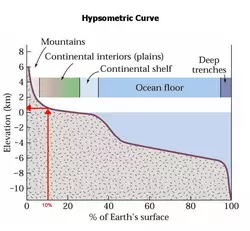# How do I read the hypsometric curve? Reading a cumulative percent graph

## An introduction to the hypsometric curve

The hypsometric curve from Marshak "Earth - Portrait of the Planet" 2nd ed. p. 37.

You may want to print a copy of the hypsometric curve to work from. Your textbook may have a slightly different version.

The hypsometric curve is the graph on the right hand side of this page. This curve is typically used to demonstrate that the Earth has two types of crust, continental and oceanic. The curve shows the percentage of the Earth's surface above any elevation. The horizontal axis, labeled "% of Earth's surface," gives the percentage while the vertical axis shows elevation above or below sea level.

Although there is other information on this plot, our focus is the dark red curve that crosses the plot. (If you aren't sure about which is the red line, you can view this movie that shows which line (Quicktime Video 3.7MB Aug21 08).) Note that the red line crosses the y-axis at about 6 km. Similarly, the line crosses the x-axis when y=-12 km and x=100%.

## How do I read the graph?

Focus on the dark red curve. Remember, there are two variables plotted on this graph (elevation and % of Earth's surface). Typically you will be given a value for one of these variables, and you will determine the value of the other. When you are given a percentage, read it on the horizontal axis and go up until you reach the curve. Then draw a line across to the vertical axis, and read the answer, the elevation above which the given percent of the Earth's surface can be found. On the other hand, when given an elevation, you draw a horizontal line to the curve, drop a line down to the bottom axis and read the percentage. This is easier to understand using an example.Let's try an example: Using the hypsometric curve, determine the minimum elevation of mountains that make up the highest 10% of the Earth's surface. Let's take it step by step, using the figure to the left (you can click on it to get a bigger version):

1. Find 10% on the horizontal axis.
2. Draw a line up from 10% until it reaches the hypsometric curve (the dark red curve).
3. Now, draw a line across to the vertical axis (at approximately 0.5 km).
4. So, you find that 10% of the Earth's surface has an elevation of 0.5 km or higher.
Determining % of Earth's surface above a given elevation

We can also figure out how much of the Earth's surface is above a certain elevation.

What percentage of the Earth's surface is above sea level.

Try this on your own first with the blank hypsometric curve above. If you get stuck, you can peek at the answer below:

Determining % of Earth's surface below a given elevation

A slightly more complicated problem is determining the portion of the Earth's surface that is below a given elevation. We need only add one step to complete these types of problems. Just subtract the percentage above from 100% to get the percentage below. In other words, if a given percentage of the Earth's surface is above a given elevation, the rest of it must be below that elevation.

Determine the % of the Earth's surface that is below sea level.

Again, try it on your own, but you can peek if you need to:

Determining % of Earth's surface between two elevations

We can also determine the amount of the Earth's surface that is between two elevations. This kind of problem involves making two determinations of % and then subtracting them from one another./

Let's try the example of determining the percentage of the Earth's surface between 5 km below sea level and 1 km below sea level.

## Where is the hypsometric graph or similar graphs are used in the geosciences?

The hypsometric curve is used when discussing
• Isostasy - buoyancy of the oceanic crust, elevation changes due to temperature changes in the crust,
• Continental and oceanic crust - determining the elevation of oceanic and continental crust, differentiating between oceanic and continental crust
• Planetary geology - the examination of other planets to look for different surfaces, the examination of planetary surfaces for evidence of plate tectonics.

In addition to these, cumulative percent graphs like the hypsometric curve are used in a wide variety of contexts both in the geosciences and in many other fields. However once you learn to interpret one, you can read them all!

Other geological contexts that use cumulative percent graphs include:

• Igneous Rocks - Igneous rock identification
• Sedimentology - Grain size distributions

OK, now go ahead and go to the sample problems.

## More help

Annanberg learner learning MATH telecourse includes instructions on distributions and representing distributions. There are sample problems and more. This is meant as a source to help students teach themselves how about distributions and representing distributions.

Continue to the sample problems.

This page was written and compiled by Dr. Eric M. Baer, Geology Program, Highline Community College,
and
Dr. Jennifer M. Wenner, Geology Department, University of Wisconsin Oshkosh.